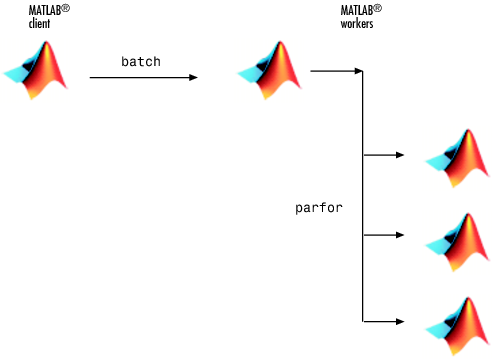Documentation

### This is machine translation

Mouseover text to see original. Click the button below to return to the English version of the page.

To view all translated materials including this page, select Country from the country navigator on the bottom of this page.

## Run Batch Parallel Jobs

### Run a Batch Job

To offload work from your MATLAB® session to run in the background in another session, you can use the `batch` command inside a script.

1. To create the script, type:

`edit mywave`
2. In the MATLAB Editor, create a `for`-loop:

```for i = 1:1024 A(i) = sin(i*2*pi/1024); end```
3. Save the file and close the Editor.

4. Use the `batch` command in the MATLAB Command Window to run your script on a separate MATLAB worker:

`job = batch('mywave')`5. The `batch` command does not block MATLAB, so you must wait for the job to finish before you can retrieve and view its results:

`wait(job)`
6. The `load` command transfers variables created on the worker to the client workspace, where you can view the results:

```load(job,'A') plot(A)```
7. When the job is complete, permanently delete its data and remove its reference from the workspace:

```delete(job) clear job```

`batch` runs your code on a local worker or a cluster worker, but does not require a parallel pool.

You can use `batch` to run either scripts or functions. For more details, see the `batch` reference page.

### Run a Batch Job with a Parallel Pool

You can combine the abilities to offload a job and run a loop in a parallel pool. This example combines the two to create a simple batch `parfor`-loop.

1. To create a script, type:

`edit mywave`
2. In the MATLAB Editor, create a `parfor`-loop:

```parfor i = 1:1024 A(i) = sin(i*2*pi/1024); end```
3. Save the file and close the Editor.

4. Run the script in MATLAB with the `batch` command. Indicate that the script should use a parallel pool for the loop:

`job = batch('mywave','Pool',3)`

This command specifies that three workers (in addition to the one running the batch script) are to evaluate the loop iterations. Therefore, this example uses a total of four local workers, including the one worker running the batch script. Altogether, there are five MATLAB sessions involved, as shown in the following diagram.5. To view the results:

```wait(job) load(job,'A') plot(A)```

The results look the same as before, however, there are two important differences in execution:

• The work of defining the `parfor`-loop and accumulating its results are offloaded to another MATLAB session by `batch`.

• The loop iterations are distributed from one MATLAB worker to another set of workers running simultaneously (`'Pool'` and `parfor`), so the loop might run faster than having only one worker execute it.

6. When the job is complete, permanently delete its data and remove its reference from the workspace:

```delete(job) clear job```